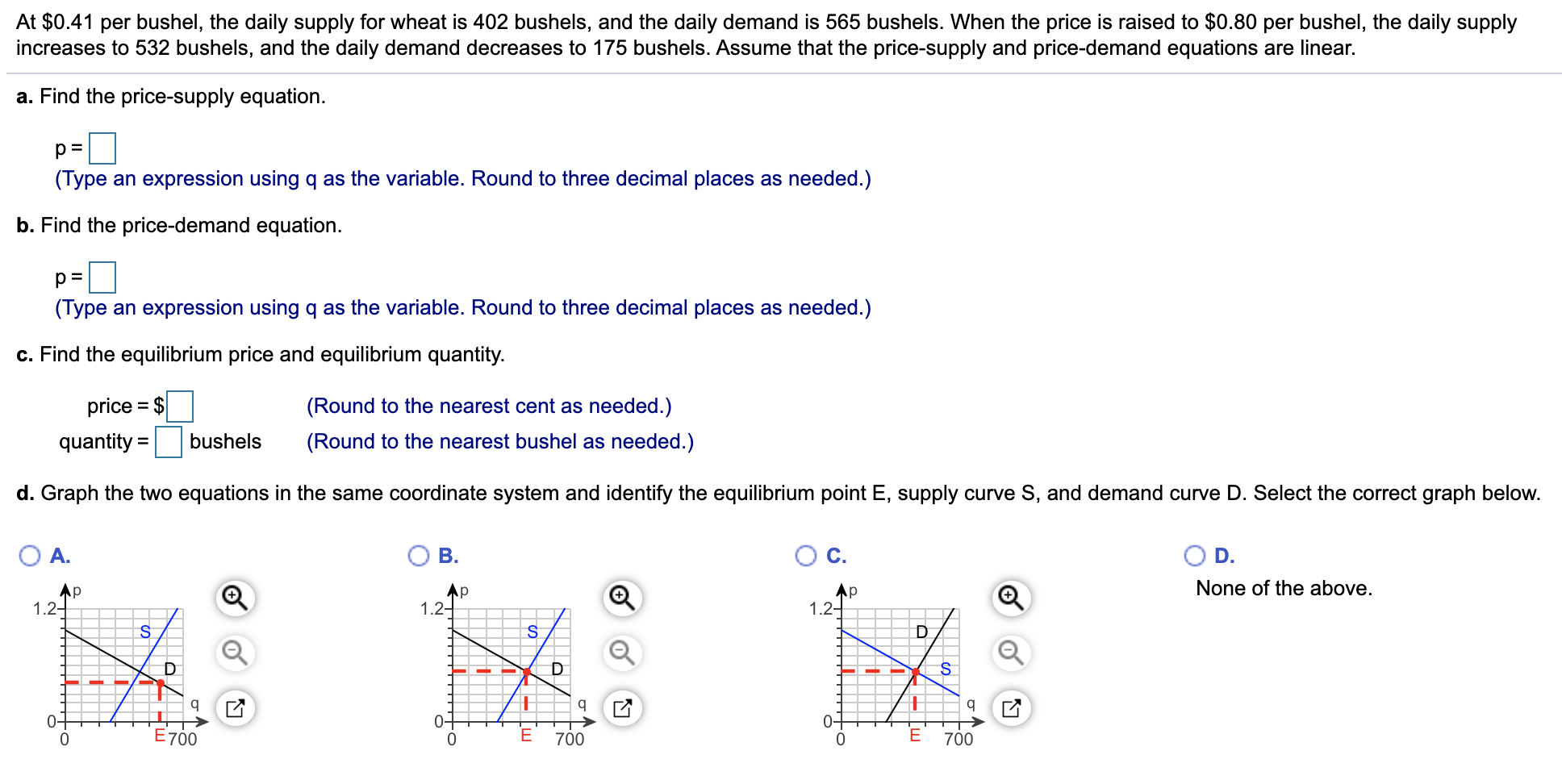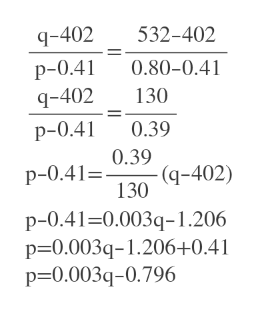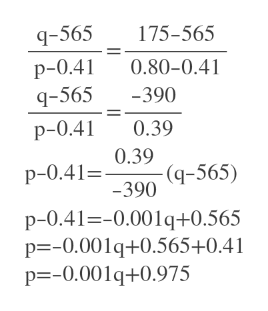# At \$0.41 per bushel, the daily supply for wheat is 402 bushels, and the daily demand is 565 bushels. When the price is raised to \$0.80 per bushel, the daily supplyincreases to 532 bushels, and the daily demand decreases to 175 bushels. Assume that the price-supply and price-demand equations are linear.a. Find the price-supply equation.p =(Type an expression using q as the variable. Round to three decimal places as needed.)b. Find the price-demand equationp =(Type an expression using q as the variable. Round to three decimal places as needed.)c. Find the equilibrium price and equilibrium quantityprice \$(Round to the nearest cent as needed.)quantitybushels(Round to the nearest bushel as needed.)d. Graph the two equations in the same coordinate system and identify the equilibrium point E, supply curve S, and demand curve D. Select the correct graph belowОс.ОА.В.D.None of the aboveAp1.2Ар1.21.2Dqq0-00-0E700700700--E

Question
1 viewshelp_outlineImage TranscriptioncloseAt \$0.41 per bushel, the daily supply for wheat is 402 bushels, and the daily demand is 565 bushels. When the price is raised to \$0.80 per bushel, the daily supply increases to 532 bushels, and the daily demand decreases to 175 bushels. Assume that the price-supply and price-demand equations are linear. a. Find the price-supply equation. p = (Type an expression using q as the variable. Round to three decimal places as needed.) b. Find the price-demand equation p = (Type an expression using q as the variable. Round to three decimal places as needed.) c. Find the equilibrium price and equilibrium quantity price \$ (Round to the nearest cent as needed.) quantity bushels (Round to the nearest bushel as needed.) d. Graph the two equations in the same coordinate system and identify the equilibrium point E, supply curve S, and demand curve D. Select the correct graph below Ос. ОА. В. D. None of the above Ap 1.2 Ар 1.2 1.2 D q q 0- 0 0- 0 E700 700 700 --E fullscreen
check_circle

star
star
star
star
star
1 Rating
Step 1

p=price and q= quantity

a)  For price - supply two coordinates are(p,q)= (0.41,402) and (0.80,532)

Then use two point formula to find the equation of the line.help_outlineImage Transcriptioncloseq-402 532-402 0.80-0.41 р-0.41 130 q-402 0.39 р-0.41 0.39 (q-402) 130 р-0.41%3. p-0.41-0.003q-1.206 p 0.003q-1.206+0.41 p-0.003q-0.796 fullscreen
Step 2

p=price and q= quantity

b)  For price - demand two coordinates are(p,q)= (0.41,565) and (0.80,175)

Then use two point formula to find the equation of the line.help_outlineImage Transcriptioncloseq-565 175-565 p-0.4 q-565 0.80-0.41 -390 p-0.41 0.39 0.39 -(q-565) -390 p-0.41= p-0.41--0.001q+0.565 p=-0.001q+0.565+0.41 p -0.001q+0.975 fullscreen
Step 3

c) To find equilibrium point set the two prices equal and solve for q.

q=443

For q=443 , ...

### Want to see the full answer?

See Solution

#### Want to see this answer and more?

Solutions are written by subject experts who are available 24/7. Questions are typically answered within 1 hour.*

See Solution
*Response times may vary by subject and question.
Tagged in

### Other